Courses
Courses for Kids
Free study material
Free LIVE classes
More

# 2- Digit by 1-Digit MultiplicationLIVE
Join Vedantu’s FREE Mastercalss

Multiplication or repeated addition is an operation through which we can add things up in a fast manner. Multiplication is done between two numbers. The numbers can be one digit or two digits or three digits. Single-digit multiplication worksheets will help you better understand multiplication.

## What are 1-Digit Numbers?

The number which represents a single digit or a single number, that number is said to be a 1-digit number. There are nine one-digit numbers from 0 to 9. The smallest one-digit number is 0 and the largest one-digit number is 9.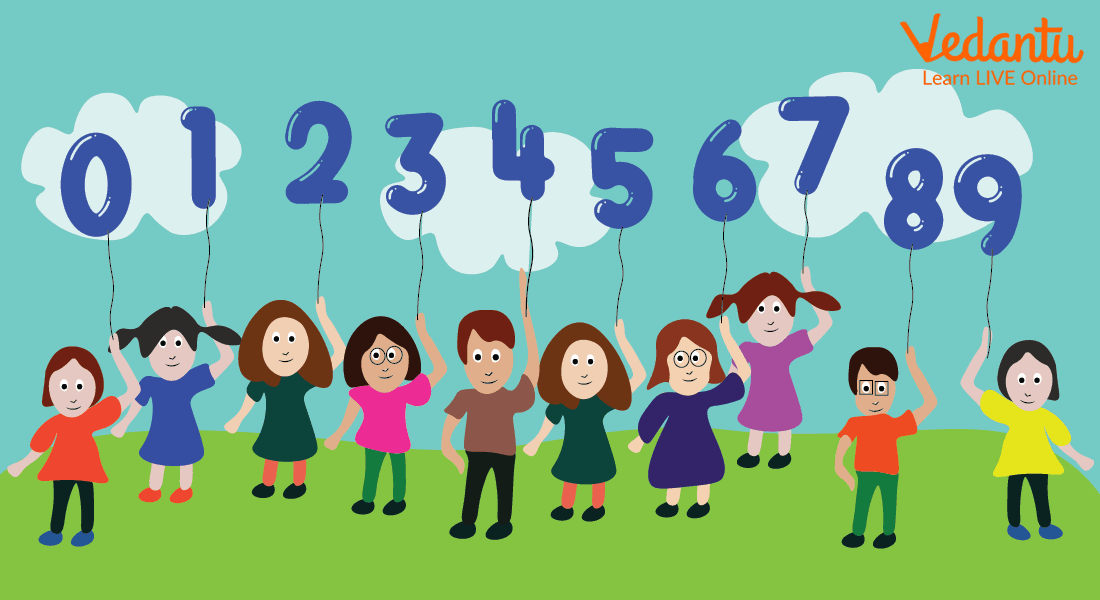1-Digit Numbers

## What are 2-Digit Numbers?

A two-digit number is formed by using two digits from 0 to 9. This 2-digit number starts from 10 and ends at 99. The smallest two-digit number is 10 and the largest two-digit number is 99.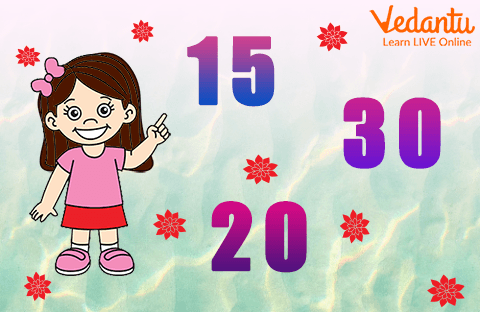2-Digit Numbers

## Multiplication of 1-Digit by 1-Digit Number

Multiplication of numbers is done by using the multiplication symbol which is a cross. The number written before the cross is called a multiplicand and the number written after is multiplied. Multiplication of 1 digit number by 1 digit number is similar to the multiplicative tables that we learnt. For example, multiply 4 by 2. Here, 4 is the multiplicand and 2 is the multiplier which gives the result as 8.

## Multiplication of 2-Digit by 1-Digit Number

Let us consider an example of 2-digit by 1-digit multiplication with no regrouping. Here, no grouping means that during multiplication, there would not be any carryover. Multiply 23 by 2. For multiplying a 2-digit by a 1-digit number, follow the following steps:

Step One: Write the largest number or the multiplicand on the top side and one digit number or the multiplier on the bottom as shown.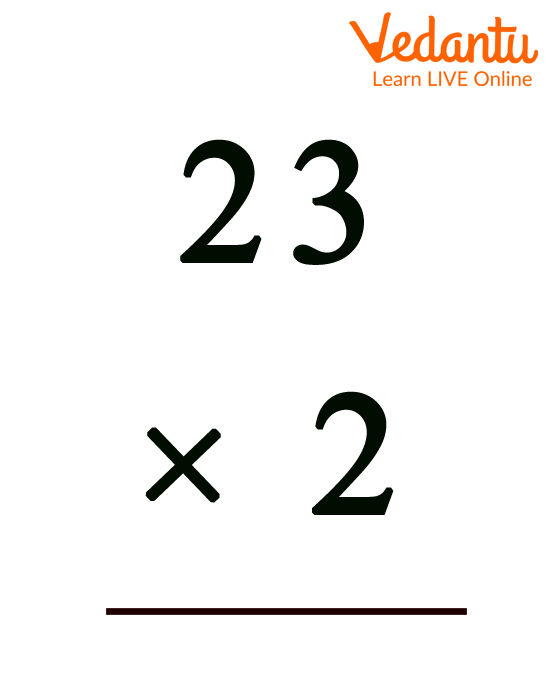Step One of Example

Step Two: Multiplying each number on the top by the bottom one-digit number, we get the result as shown in the below image.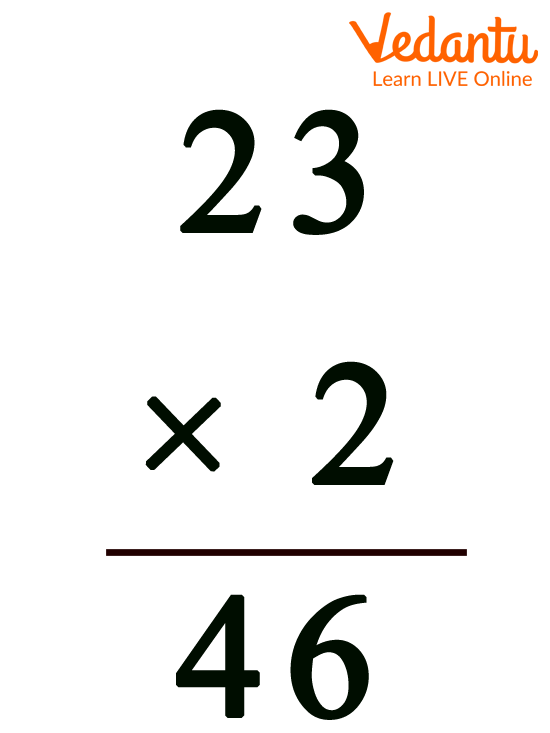Step Two of Example

Step Three: The answer which will come is the product of the number.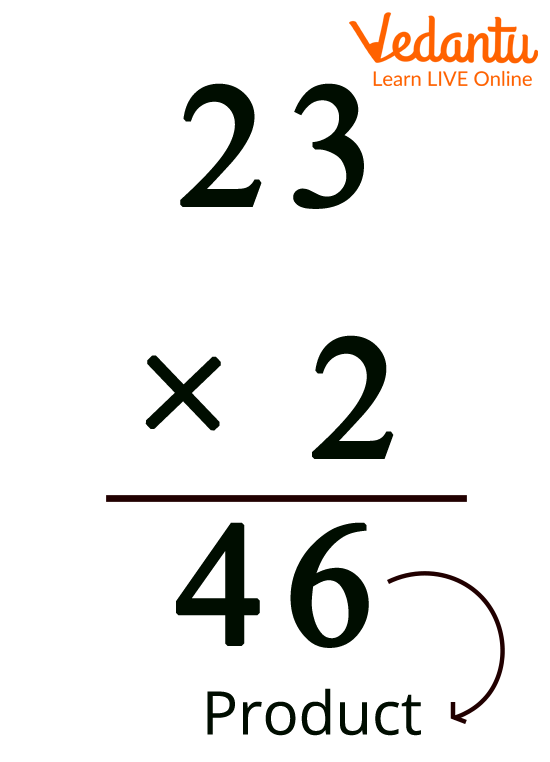Step Three of Example

## 2-Digit by 1-Digit Multiplication Worksheets

1. Multiply 43 by 3.

Ans: Step One: Write the multiplicand and the multiplier.

Step Two: multiply each digit of the multiplicand by the multiplier.

Step Three: The result obtained after multiplication is the product.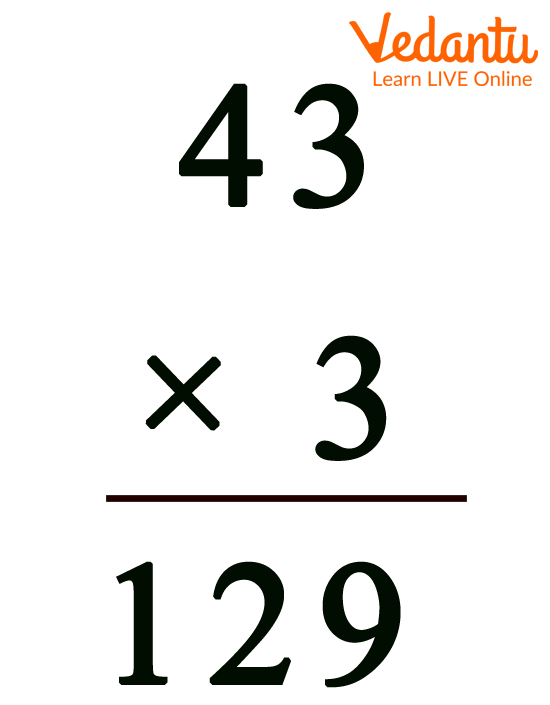Product of 43 by 3 is 129

2. Find the product of 60 and 9.

Ans: Step One: Write the multiplicand and the multiplier.

Step Two: Multiply each digit of the multiplicand by the multiplier.

Step Three: The result obtained after multiplication is the product.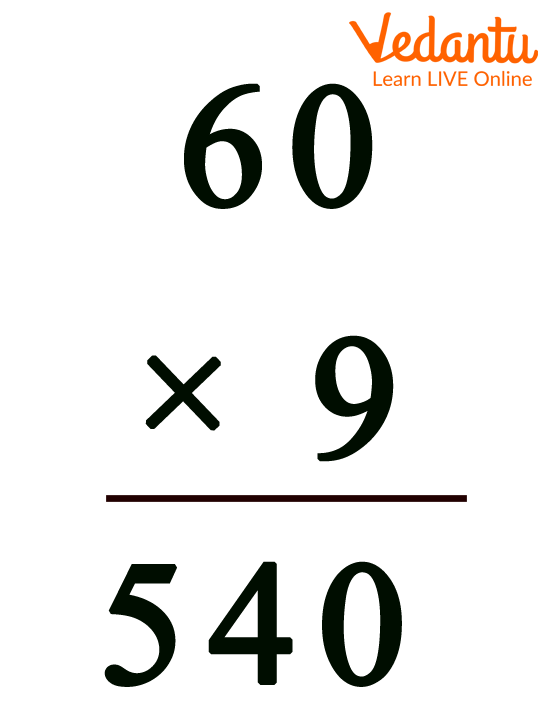The Product of 60 by 9 is 540

3. If 91 and 2 are multiplied by each other, then what will be the answer?

Ans: Step One: Write the multiplicand and the multiplier.

Step Two: Multiply each digit of the multiplicand by the multiplier.

Step Three: The result obtained after multiplication is the product.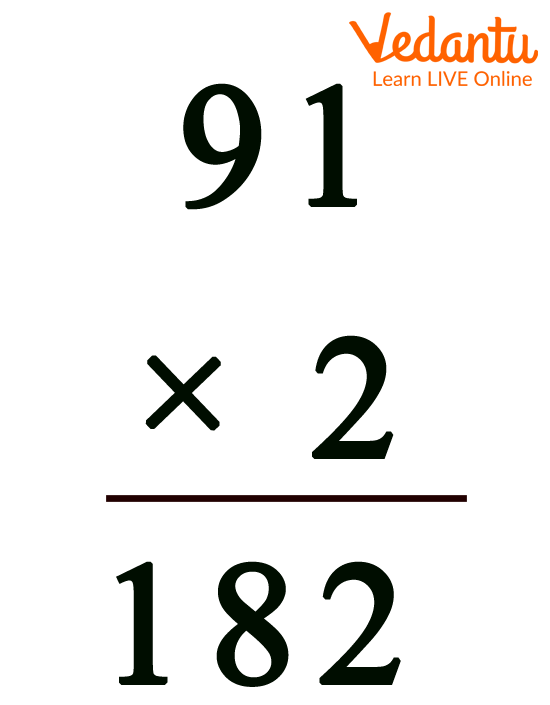The Answer to Multiplying 91 by 2 will be 182

## Conclusion

Multiplication is a mathematical operation that provides the fundamental concept of repeated addition of the same integer. In our daily lives, we frequently employ multiplication. For example, we may calculate the price of things based on the rate per amount, discover the proper quantity of an ingredient to use in cooking, calculate the value of numerous items when the value of one item is known and so on.

Last updated date: 21st Sep 2023
Total views: 92.4k
Views today: 0.92k

## FAQs on 2- Digit by 1-Digit Multiplication

1. What are the multiplication numbers called?

The integers to be multiplied are commonly referred to as "factors." The "multiplicand" is the number to be multiplied, and the "multiplier" is the number by which it is multiplied.

2. Where does multiplication function?

Multiplication allows us to swiftly determine the total number of objects. We'll consider the number of equal portions and the amounts of items in each group.

3. By what number you will multiply any number to get the number itself and zero?

On multiplying any number by 1, you get the same number itself as a product and on multiplying any number by 0, the product will always be equal to zero.

4. How will you describe the multiplication operation?

Multiplication can be defined as the repeated addition of a number. For example, multiplication of 3 and 4 means adding the number 4 three times or adding the number 3 four times. There are two terms used in multiplication that are a factor (a number that is to be multiplied) and product (the answer to the multiplication).